# 气体传感器性能准确快速评价方法研究 Research on Accurate and Fast Evaluation De-vice Using for Gas Sensor Performance

DOI: 10.12677/JSTA.2020.83009, PDF, HTML, XML, 下载: 151  浏览: 449  科研立项经费支持

Abstract: In this paper, an evaluation system using for gas sensor performance has been constructed, which comprised with three parts: a multicomponent dynamic gas distribution unit, a micro test chamber as core and a data analysis unit. The simulations of gas flow states for the internal micro test chamber were employed via the standard k-ε equation (k is the turbulent kinetic energy, ε is the dissipation rate) in ANSYS FLUENT software based on inner chamber structure modeling by SolidWorks software. The effect of flow velocity on three gas sensors performance and the tightness of the micro test chamber were investigated. The response performance of Sensor W and Sensor Z was exhibited via the as-constructed evaluation device by using methanal and toluene as target components. In contrast to the nominal value, Sensor W has obtained more accurate and objective sensing properties, i.e. the lower detection limit (0.1 ppm) with the corresponding sensitivity ((Rg/Ra, 0.926). Sensor Z has been demonstrated excellent sensing response towards methanal and toluene, range from 500 ppb - 10 ppm and 5 ppb - 300 ppb, respectively. Simultaneously, the response and recovery times of Sensor Z for methanal and toluene at 5 ppb and 500 ppb respectively, were accurately measured. As the results, the evaluation system for gas sensor performance as-constructed in this paper has the advantages of stable internal gas flow field, uniform gas concentration distribution and satisfied gas tightness as well as the evaluation capability for ultrasensitive and fast gas sensor.

1. 引言

2. 实验部分

2.1. 微型测试舱及测试系统的设计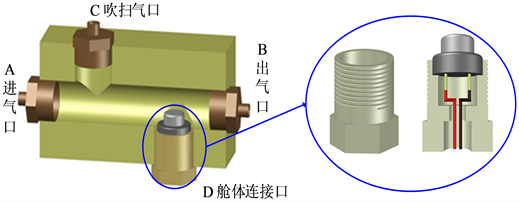Figure 1. Micro test chamber cross-section and sensor holder structures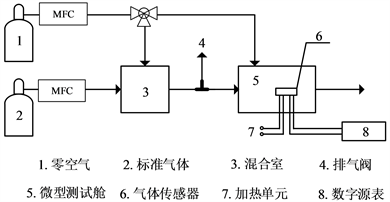Figure 2. Schematic diagram of test system

${C}_{T}=\frac{{Q}_{S}}{{Q}_{Z}+{Q}_{S}}{C}_{S}$

2.2. 测试舱内部气流场模拟

$\frac{\partial }{\partial t}\left(\rho k\right)+\frac{\partial }{\partial {x}_{i}}\left(\rho k{u}_{i}\right)=\frac{\partial }{\partial {x}_{j}}\left[\left(\mu +\frac{{\mu }_{t}}{{\sigma }_{k}}\right)\frac{\partial k}{\partial {x}_{j}}\right]+{G}_{k}+{G}_{b}-\rho \epsilon -{Y}_{M}+{S}_{k}$ (1)

$\frac{\partial }{\partial t}\left(\rho \epsilon \right)+\frac{\partial }{\partial {x}_{i}}\left(\rho \epsilon {u}_{i}\right)=\frac{\partial }{\partial {x}_{j}}\left[\left(\mu +\frac{{\mu }_{t}}{{\sigma }_{\int }}\right)\frac{\partial \epsilon }{\partial {x}_{j}}\right]+{C}_{1\epsilon }\frac{\epsilon }{k}\left({G}_{k}+{C}_{3\epsilon }{G}_{b}\right)-{C}_{2\epsilon }\rho \frac{{\epsilon }^{2}}{k}+{S}_{\epsilon }$ (2)

2.3. 评价装置的构建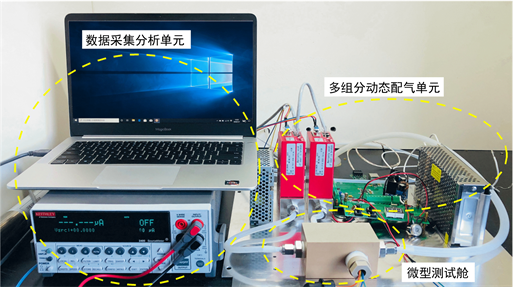Figure 3. Evaluation device for performances of gas sensor

3. 微型测试舱性能分析

3.1. 测试舱气体流场分析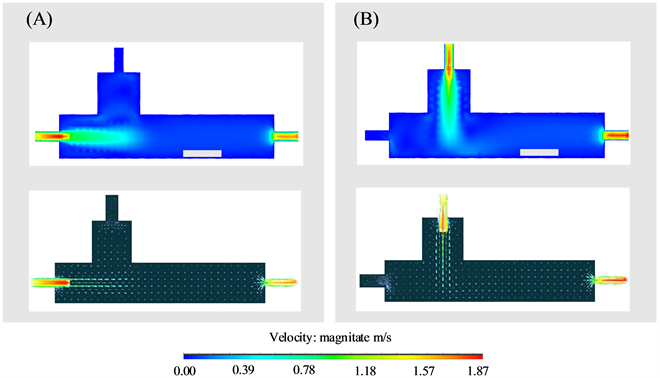Figure 4. The gas velocity cloud and vector diagrams for (A) Inlet and (B) Purge inletof the internal test chamber

3.2. 测试舱气密性检验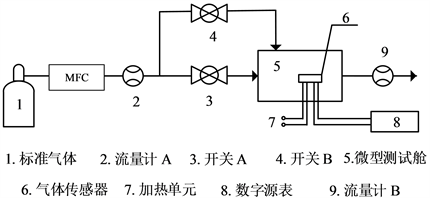Figure 5. Schematic diagram of test chamber air tightness test

$\frac{泄露率{V}_{L}}{供气率{V}_{S}}\le 5%$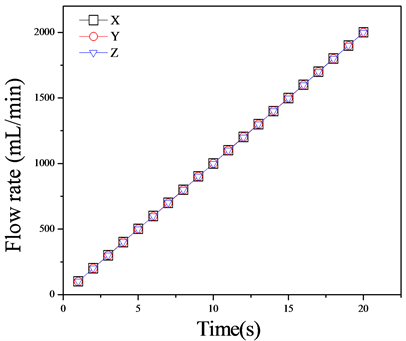Figure 6. Comparison of test chamber air inlet flow with air outlet flow

3.3. 测试舱应用效果分析

3.3.1. 入口气流对传感器基线影响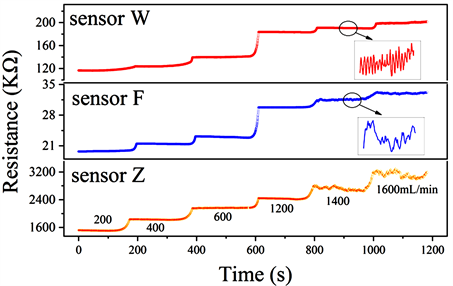Figure 7. Response base line under different inlet gas flow rates

3.3.2. 测试舱对传感器性能评价

Sensor W对甲苯的标称检测下限为10 ppm，灵敏度(Rg/Ra)为0.52，本研究构建的评价系统对Sensor W性能评价结果如图8所示，甲苯检测下限为0.1 ppm，灵敏度(Rg/Ra)为0.926，检测范围为0.1 ppm~100 ppm测试范围，与标称值相比，本次测试Sensor W的性能指标，包括灵敏度、检测限及检测范围，均有所提高。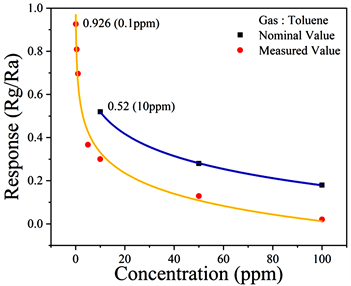Figure 8. Comparison of mearsured value with nominal value for sensor W sensitivity

Sensor Z在系统中的性能评价结果，在最佳工作温度下，Sensor Z对不同浓度的甲苯和甲醛的灵敏度响应如图9(A)所示，在500 ppb~10 ppm范围内，随着甲醛浓度的阶跃式增加，Sensor Z的响应稳步上升；同样，在5 ppb~300 ppb范围内，Sensor Z的灵敏度随着甲苯浓度增加而上升。图9(B)为Sensor Z对500 ppb甲醛和5 ppb甲苯的动态响应恢复曲线，对甲醛和甲苯的响应时间(T90)分别为11 s和36 s，恢复时间(T10)分别为2 s和9 s。Sensor Z本身对多种还原性气体具有良好的灵敏度和响应恢复特性，该系统能够满足其低浓度动态条件下的气敏特性分析。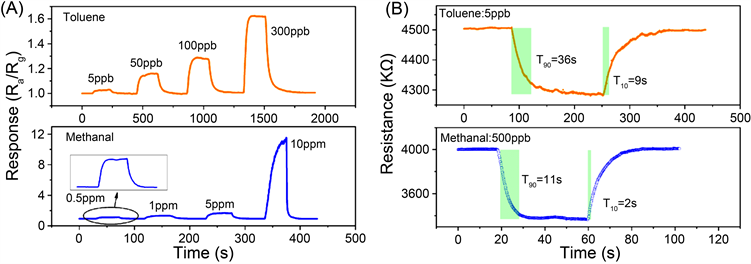Figure 9. (A) Sensor Z对不同浓度甲醛和甲苯的灵敏度响应曲线；(B) Sensor Z对500 ppb甲醛和5 ppb甲苯的动态响应恢复曲线

4. 结论

NOTES

*第一作者。

#通讯作者。

  Seiyama, T., Kato, A., Fujishi, K., et al. (1962) A New Detector for Gaseous Components Using Semiconductive Thin Films. Analytical Chemistry, 34, 1502-1503. https://doi.org/10.1021/ac60191a001  Sun, Y.J., Wang, Z.P., Wang, W.D., et al. (2019) Electrospinning Preparation of Pd@Co3O4-ZnO Composite Nanofibers and Their Highly En-hanced VOC Sensing Properties. Materials Research Bulletin, 109, 255-264. https://doi.org/10.1016/j.materresbull.2018.10.001  Wei, S.H., Yu, Y., Zhou, M., et al. (2010) CO Gas Sens-ing of Pd-Doped ZnO Nanofibers Synthesized by Electrospinning Method. Materials Letters, 64, 2284-2286. https://doi.org/10.1016/j.matlet.2010.07.038  Kolhe, P.S., Koinkar, P.M., Maiti, N., et al. (2017) Synthesis of Ag Doped SnO2 Thin Films for the Evaluation of H2S Gas Sensing Properties. Physica B Condensed Matter, 524, 90-96. https://doi.org/10.1016/j.physb.2017.07.056  Sun, Y.G., Wang, H.H. and Xia, M. (2008) Single-Walled Car-bon Nanotubes Modified with Pd Nanoparticles: Unique Building Blocks for High-Performance, Flexible Hydrogen Sensors. Journal of Physical Chemistry C, 112, 1250-1259. https://doi.org/10.1021/jp076965n  Boon-Brett, L., Bousek, J., Black, G., et al. (2010) Identifying Performance Gaps in Hydrogen Safety Sensor Technology for Automotive and Stationary Applications. International Journal of Hy-drogen Energy, 35, 373-384. https://doi.org/10.1016/j.ijhydene.2009.10.064  Boon-Brett, L., Black, G., Moretto, P., et al. (2010) A Com-parison of Test Methods for the Measurement of Hydrogen Sensor Response and Recovery Times. International Journal of Hydrogen Energy, 35, 7652-7663. https://doi.org/10.1016/j.ijhydene.2010.04.139  Jang, J.S., Qiao, S., Choi, S.J., et al. (2017) Hollow Pd-Ag Composite Nanowires for Fast Responding and Transparent Hydrogen Sensors. ACS Applied Materials & Interfaces, 9, 39464-39474. https://doi.org/10.1021/acsami.7b10908  Barsan, N., Simion, C., Heine, T., et al. (2010) Mod-eling of Sensing and Transduction for p-Type Semiconducting Metal Oxide Based Gas Sensors. Journal of Elec-troceramics, 25, 11-19. https://doi.org/10.1007/s10832-009-9583-x  Li, T.Y., Chianga, S.J., Liaw, B.J., et al. (2011) Catalytic Oxidation of Benzene over CuO/Ce1−xMnxO2 Catalysts. Applied Catalysis B: Environmental, 103, 143-148. https://doi.org/10.1016/j.apcatb.2011.01.020  Tobias, P., Mårtensson, P., Göras, A., et al. (1999) Moving Gas Outlets for the Evaluation of Fast Gas Sensors. Sensors and Actuators B: Chemical, 58, 389-393. https://doi.org/10.1016/S0925-4005(99)00120-3  Sawaguchi, N., Nishibori, M. and Tajima, K. (2006) Practical Test Methods for Hydrogen Gas Sensor Response Characterization. Electrochemistry, 74, 315-320. https://doi.org/10.5796/electrochemistry.74.315  ISO 16000-6-2011. Indoor Air-Part 6: Determination of Vola-tile Organic Compounds in Indoor and Test Chamber Air by Active Sampling on Tenax TA Sorbent, Thermal Desorption and Gas Chromatography Using MS or MS-FID.  付国太, 刘洪军, 张柏, 等. PEEK的特性及应用[J]. 工程塑料应用, 2006(10): 69-71.  朱斌, 殷晨波, 陶春旻, 等. 基于恒流配气方式的微气体传感器测试系统研究[J]. 仪器仪表学报, 2011, 32(12): 2681-2687.  马宏伟, 韩根亮, 徐武德, 等. 高精度气敏特性动态测试装置的设计与实现[J]. 自动化仪表, 2018, 39(6): 13-17.  Hashim, U., Zaki, M., Arshad, M.K.M., et al. (2015) Develop-ment of Effectively Test Chamber for SnCl2 Gas Detection Sensor. 2015 2nd International Conference on Biomedical Engineering, Penang, 30-31 March 2015, 1-4. https://doi.org/10.1109/ICoBE.2015.7235900  李剑光, 李赵相, 唐光磊, 等. 室内环境测试舱性能指标及测试方法的探讨[J]. 山西建筑, 2016, 42(10): 201-202.  全国家具标准化技术委员会. GB/T 31107-2014家具中挥发性有机化合物检测用气候舱通用技术条件[S]. 北京: 中国标准出版社, 2014.